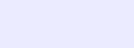# Momentum

Physical quantity that describes the quantity of motion in a body is called momentum.
The momentum of a moving body is defined as
“the product of mass and velocity of a moving body is called linear momentum”
Mathematically,Momentum is a vector quantity and its direction is the same as that of velocity.

Explanation
Momentum is that property of a moving body which determines how much effort is required to accelerate or stop a body. Hence, it may also be termed as quantity of motion of a body. From various observations it is concluded that greater effort is required to stop a body if it possesses either greater mass or greater velocity or both.
Units Of Momentum
In S.I. system : NS [1 NS = 1 kg m/s]
In C.G.S. system : Dyne.S
In F.P.S. system : Lb.S
Dimensions Of Momentum
The dimension of momentum is [MLT-1].